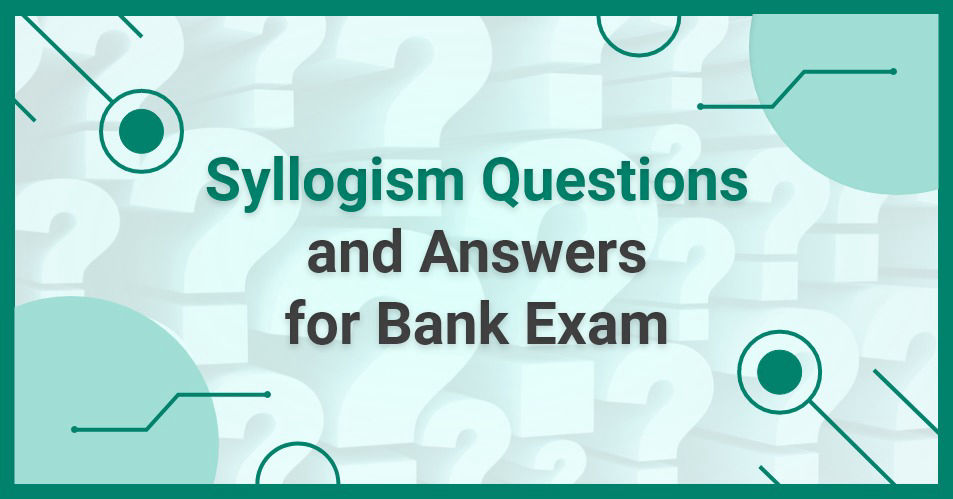• Save

# Syllogism Questions and Answers for Bank ExamLogical Reasoning is said to be quite tricky but if the aspirants have prepared well in this section then they can get good scores in the examination. syllogism is an important topic of logical Reasoning which covers approx 5 questions related to the bank exam. Today this article, provided important questions which are based on syllogism. Let's start practice below -

## Banking Related Syllogism Questions

Directions: In each question below are given some statements followed by two conclusions numbered I and II. You have to take the given statements to be true even if they seem to be at variance with commonly known facts. Read all the conclusions and then decide which of the given conclusions logically follows/follow from the given statements, disregarding commonly known facts.

Q :

Statements:

All carbon are oxygen.

All Nitrogen are carbon.

Some oxygen are Sulphur.

Conclusions:

I. All Nitrogen being Sulphur is a possibility.

II. All Nitrogen are not oxygen.

(A) If only conclusion I follow.

(B) If only conclusion II follow.

(C) If neither conclusion I nor conclusion II follows.

(D) If both the conclusions follow.

(E) If either conclusion I or conclusion II follows.

Directions: In each question below are given some statements followed by two conclusions numbered I and II. You have to take the given statements to be true even if they seem to be at variance with commonly known facts. Read all the conclusions and then decide which of the given conclusions logically follows/follow from the given statements, disregarding commonly known facts.

Q :

Statements:

Some frooti are Maaza.

No Maaza is slice.

All slice are fanta.

Conclusions:

I. Some frooti are definitely not slice.

II. Some fanta are definitely not Maaza.

(A) If only conclusion I follow.

(B) If only conclusion II follow.

(C) If neither conclusion I nor conclusion II follows.

(D) If both the conclusions follow.

(E) If either conclusion I or conclusion II follows.

Directions: In each question below are given some statements followed by two conclusions numbered I and II. You have to take the given statements to be true even if they seem to be at variance with commonly known facts. Read all the conclusions and then decide which of the given conclusions logically follows/follow from the given statements, disregarding commonly known facts.

Q :

Statements:

All shirts are skirts.

No skirt is top.

All tops are kurta.

Conclusions:

I. All shirts are kurta

II. Some kurta are skirts

(A) If only conclusion I follow.

(B) If only conclusion II follow.

(C) If neither conclusion I nor conclusion II follows.

(D) If both the conclusions follow.

(E) If either conclusion I or conclusion II follows.

Directions: In each question below are given some statements followed by two conclusions numbered I and II. You have to take the given statements to be true even if they seem to be at variance with commonly known facts. Read all the conclusions and then decide which of the given conclusions logically follows/follow from the given statements, disregarding commonly known facts.

Q :

Statements :      Some balls are bats.

No bat is a wicket.

Conclusions :      I. Some wickets are not ball.

II. All wickets being ball is a possibility.

(A) if only conclusion I follows.

(B) if only conclusion II follows.

(C) if either conclusion I or conclusion II follows.

(D) if neither conclusion I nor conclusion II follows.

(E) if both conclusion I and conclusion II follow.

Directions: In each question below are given some statements followed by two conclusions numbered I and II. You have to take the given statements to be true even if they seem to be at variance with commonly known facts. Read all the conclusions and then decide which of the given conclusions logically follows/follow from the given statements, disregarding commonly known facts.

Q :

Statements : No water is air.

No fire is water.

Conclusions : I.    No air is fire.

II.   All fire is air.

(A) if only conclusion I follows.

(B) if only conclusion II follows.

(C) if either conclusion I or conclusion II follows.

(D) if neither conclusion I nor conclusion II follows.

(E) if both conclusion I and conclusion II follow.